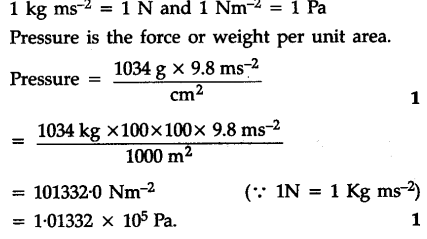# Pressure is determined as force per unit area of the surface. The SI unit of pressure, pascal is as shown below:

Pressure is determined as force per unit area of the surface. The SI unit of pressure, pascal is as shown
below:
1 Pa = 1 N\${{m}^{-2}}\$
if the mass of air at sea level is 1034 g\$c{{m}^{-2}}\$ calculate the pressure in pascal.